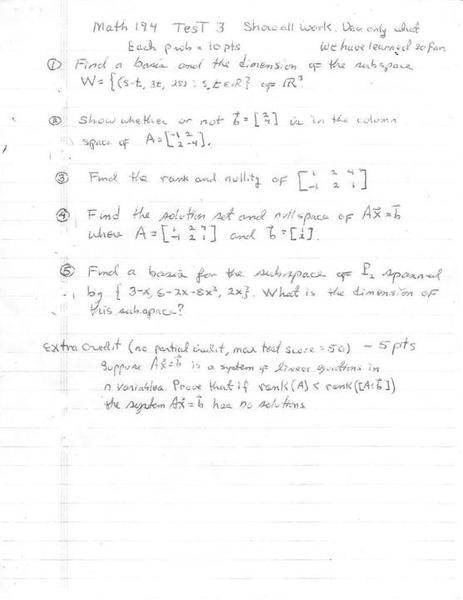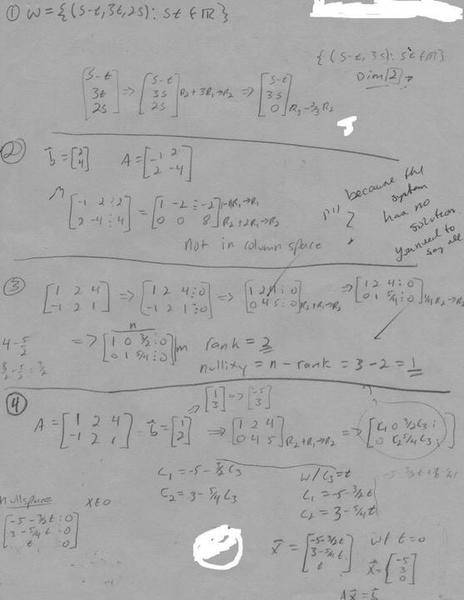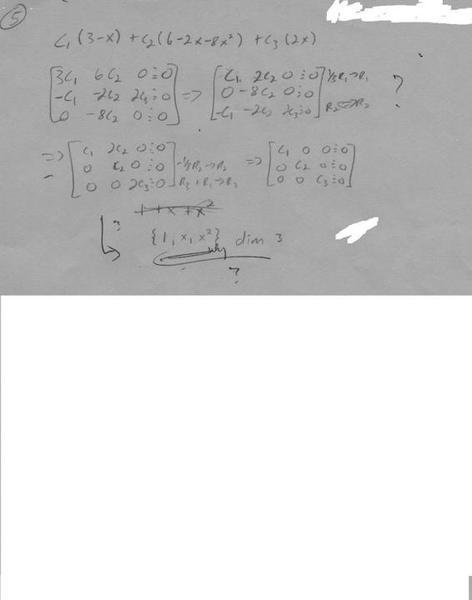# Basis and dimension: Need help finding my mistakes

## Homework Statement

He made some notes, but I'm still confused.## The Attempt at a SolutionMark44
Mentor
What specifically do you have questions on?

Well like number one. he gave me 3 out of 10 points.

Now that I look at it, since its in R3, its dimension should be 3 ya? I put two because of the s and t but that doesn't really seem to make much sense to me now..

Did I find a basis in number one?

Or like in number two. I thought I did show b is not in the column space. How much farther do I have to go and what could I have done?

The only problem I got correct was number three.

What did I do wrong in problem four? I know I didn't find the nullspace, how would I have gone about that? Does the other work look ok up to finding the nullspace?

And problem five, I thought I found the basis, but I guess I didn't.

vela
Staff Emeritus
Homework Helper
Well like number one. he gave me 3 out of 10 points.

Now that I look at it, since its in R3, its dimension should be 3 ya? I put two because of the s and t but that doesn't really seem to make much sense to me now..

Did I find a basis in number one?
No, you didn't. When asked to find a basis for W, you need to provide a set of linearly independent vectors that spans W. The number of vectors in that set is the dimension.

Mark44
Mentor
Or like in number two. I thought I did show b is not in the column space. How much farther do I have to go and what could I have done?
You could have explained what the bottom row of your augmented matrix meant; namely that there are no solutions for the equation 0x + 0y = 8, which means that <2, 4> is not in the column space of the matrix.

A simpler approach that requires no computation is to recognize that the two columns in the matrix are multiples of each other, so the column space is the set of multiples of <-1, 2>. The vector <2, 4> is not a multiple of <-1, 2> so is not in the column space.
The only problem I got correct was number three.

What did I do wrong in problem four? I know I didn't find the nullspace, how would I have gone about that? Does the other work look ok up to finding the nullspace?
Find the vectors x such that Ax = 0. Your work after the matrix
[1 2 4]
[0 4 5]

is incorrect. There are errors in pretty much everything after that.
And problem five, I thought I found the basis, but I guess I didn't.
You found a basis, but the instructor wanted some explanation of why {1, x, x^2} is a basis for the given subspace.

The solution given by your last matrix is c1 = c2 = c3 = 0. This implies that the three functions are linearly independent, and there are three of them, so they are a basis for the subspace. The functions {1, x, x^2} are also a basis, but I'm guessing that the instructor wanted you to say that the three given functions were linearly independent and spanned P2.

I probably should of taken this class during the 18 week semester and not the six week :/

So I think I have a severe problem when it comes to understanding what a basis is. I guess I can understands its mathematical definitions, but what is a basis? Whats the point, what does it do..

No, you didn't. When asked to find a basis for W, you need to provide a set of linearly independent vectors that spans W. The number of vectors in that set is the dimension.

So for problem one, should I have chosen t to be 0 and find the resulting coefficient matrix, then the same for s = 0? Then I have two (what are they? sets of vectors?), one multiplied by s, the other by t.

Like s(1,0,2) + t(-1,3,0)? I get so confused sometimes. Maybe its the book or the instructor but I thought linear algebra was supposed to be easier then vector calculus!! lol.. What does it mean in words, or english, when a set of vectors span a vector space W.

You could have explained what the bottom row of your augmented matrix meant; namely that there are no solutions for the equation 0x + 0y = 8, which means that <2, 4> is not in the column space of the matrix.

A simpler approach that requires no computation is to recognize that the two columns in the matrix are multiples of each other, so the column space is the set of multiples of <-1, 2>. The vector <2, 4> is not a multiple of <-1, 2> so is not in the column space.

Hmm ok I see what you're saying.. And as for getting points off, the only reason I missed points was because he wanted me to say there were no solutions? Isn't that implied? I have 0+0=8 which doesn't make sense. Why do I have to use English when math says it? At least I wrote the conclusion I came to. It only asked to show. Am I being unreasonable here? He took off three points for that, out of ten.

Mark44
Mentor
frozenguy said:
Hmm ok I see what you're saying.. And as for getting points off, the only reason I missed points was because he wanted me to say there were no solutions? Isn't that implied? I have 0+0=8 which doesn't make sense. Why do I have to use English when math says it? At least I wrote the conclusion I came to. It only asked to show. Am I being unreasonable here? He took off three points for that, out of ten.
The question was posed in English, so the answer to it should also be in English. The math is the tool whereby you reach your conclusion. The notes your instructor wrote for this problem should give you a good idea about what he was looking for; namely that <2, 4> is not in the column space plus some justification that shows why you reached that conclusion.

Also, this problem is testing your understanding of what column space means. As I mentioned before, it could have been done with no matrix computation.

vela
Staff Emeritus
Homework Helper
I probably should of taken this class during the 18 week semester and not the six week :/

So I think I have a severe problem when it comes to understanding what a basis is. I guess I can understands its mathematical definitions, but what is a basis? Whats the point, what does it do.
I get so confused sometimes. Maybe its the book or the instructor but I thought linear algebra was supposed to be easier then vector calculus!! lol.. What does it mean in words, or english, when a set of vectors span a vector space W.
When a set of vectors {v1, v2, ..., vn} spans a vector space W, it just means you can write any vector x from W as a linear combination of the vectors. In other words, you could solve the equation

x = c1v1+c2v2+...+cnvn

for c1, c2, ..., cn and find at least one solution. If, in addition, the vectors {v1, v2, ..., vn} are linearly independent, there is only one solution, and we'd say they form a basis of W.

So what's the motivation for using a basis? Say you have a linear transformation T on vector space W, and {v1, v2, ..., vn} is a basis of W. Then for x in W, we can write

x = c1v1+c2v2+...+cnvn

and if now calculate T(x), we get

T(x) = c1T(v1)+c2T(v2)+...+cnT(vn)

What this means is we can understand what T does if we know how it acts on just the basis vectors. This leads to the reason you'd use one basis over another. You choose the basis where it's easy to describe what T does.
So for problem one, should I have chosen t to be 0 and find the resulting coefficient matrix, then the same for s = 0? Then I have two (what are they? sets of vectors?), one multiplied by s, the other by t.

Like s(1,0,2) + t(-1,3,0)?
In problem 1, you were given what the elements of W look like. You could do what you said above or just use regular vector manipulations:

$$\vec{w} = \begin{pmatrix}s-t \\ 3t \\ 2s\end{pmatrix} = \begin{pmatrix}s \\ 0 \\ 2s\end{pmatrix} + \begin{pmatrix}-t \\ 3t \\ 0\end{pmatrix} = s\begin{pmatrix}1 \\ 0 \\ 2\end{pmatrix} + t\begin{pmatrix}-1 \\ 3 \\ 0\end{pmatrix}$$

So you have that elements of W can be expressed as a linear combination of (1,0,2) and (-1,3,0), i.e. W is spanned by (1,0,2) and (-1,3,0). If you can show that these two vectors are linearly independent, then you know they also form a basis of W.

vela
Staff Emeritus